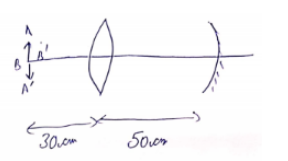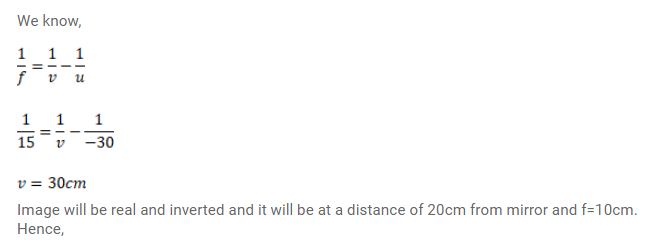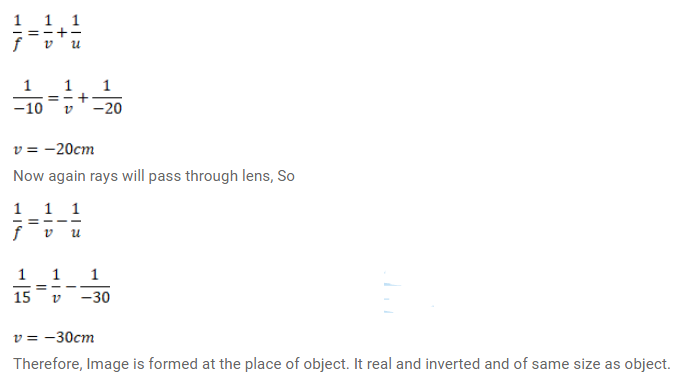# A converging lens of focal lengthQuestion:

A converging lens of focal length $15 \mathrm{~cm}$ and a converging mirror of focal length $10 \mathrm{~cm}$ are placed $50 \mathrm{~cm}$ apart. If a pin of length $2 \mathrm{~cm}$ is placed $30 \mathrm{~cm}$ from the lens farther away from the mirror, where will the final image form and what will be the size of the final image?

Solution: